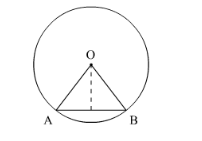# A sector is cut-off from a circle of radius 21 cm.Question:

A sector is cut-off from a circle of radius 21 cm. The angle of the sector is 120°. Find the length of its arc and the area.

Solution:

We know that the arc length / and area $A$ of a sector of circle at an angle $\theta$ of radius $r$ is given by $l=\frac{\theta}{360^{\circ}} \times 2 \pi r$ and angle $A=\frac{\theta}{360^{\circ}} \pi r^{2}$.

Let OAB is the given sector.

It is given that $O A=21 \mathrm{~cm}$ and angle $\angle A O B=120^{\circ}$.Now using the value of r and θ, we will find the value of l and A,

Arc length,

$l=\frac{120^{\circ}}{360^{\circ}} \times 2 \times \frac{22}{7} \times 21 \mathrm{~cm}$

$=44 \mathrm{~cm}$

Area of sector,

$A=\frac{120^{\circ}}{360^{\circ}} \times \frac{22}{7} \times 21 \times 21$

$=462 \mathrm{~cm}^{2}$